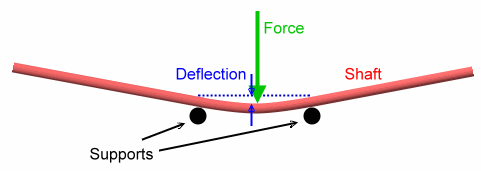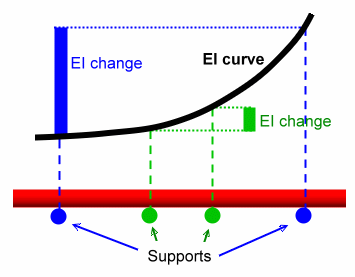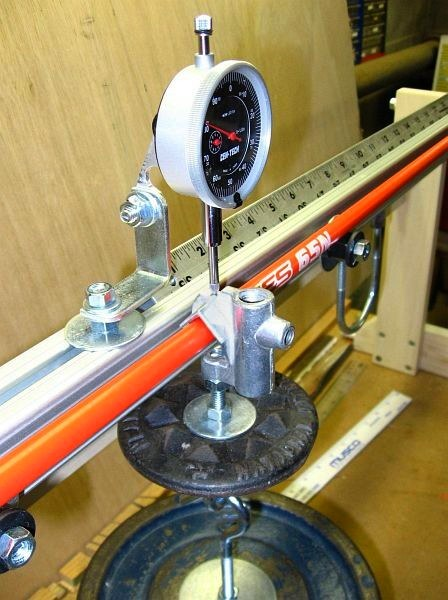# EI Machine to Profile Golf Shafts

Dave Tutelman -- November 14, 2008

The engineering description of a flex profile is the variation of EI along the shaft. EI is a structural term, the abbreviation of "E times I", where:
• E stands for "modulus of elasticity", a measure of the stiffness of the material. In particular, it says how much force it takes to stretch the material a given amount. Steel would have a very high E, and rubber a very low E. (Do not confuse elasticity with strength. Strength is the force it takes to damage the material, not to stretch it.)
• I stands for "area moment of inertia", a measure of stiffness of the cross sectional geometry of the shaft. Thicker walls or a bigger diameter make for a higher I.
This is different from the way clubfitters think of shaft profiles. When you use a frequency meter or a NeuFinder to measure a profile, each measurement is a weighted average of stiffness over a section of the shaft from the tip to some some point X, and you vary X to make a graph of the profile. EI measures the local stiffness at X, not an average from X to the tip.

## How it worksIf you support a beam (say, a golf shaft) at two points and apply a force halfway between those points, you will deflect (bend) the beam. It is pretty easy to calculate how much the beam flexes. The well-known formula for deflection at the middle, where the force is applied, is
 y = FL3 48 EI
where:
• y is the deflection
• F is the force
• L is the length between the supports
So the way to measure the EI along a shaft is to support part of it between two points and do one of two things:
1. Either apply a force in the middle between the supports, and measure the deflection, or
2. Deflect the middle a known amount, and measure the force required to do it.
Then you compute EI by solving the equation above:
 EI = FL3 48 y
What is an EI profile? The stiffness of a golf shaft varies along its length; the EI can easily be 3-4 times higher at the butt than at the tip. An EI profile is a graph of the EI over the length of the shaft. The way you measure it is to go through the steps above (apply force, measure deflection, and compute EI) for a series of points along the length of the shaft, then make a graph of those measurements.The formula works well if the stiffness doesn't vary much over the length of shaft between the supports. But the EI may change quickly enough that it can vary significantly even in the short distance between the supports. If EI varies too much between the supports, the formula loses accuracy. In the diagram, the close-together (green) set of supports does not see too much change in EI, while the farther-apart (blue) pair of supports sees a substantial change. If we consider the measured EI as the EI halfway between the supports, the green is obviously more accurate.

So the trick is to find a combination of a force and a distance between supports so that:
1. You are able to deflect the shaft enough to measure the deflection and force with some precision (implies a big force or a big distance).
2. The distance is small enough so that the EI is fairly constant between the supports (implies a small distance).
3. You don't damage the shaft (implies a small force).
Obviously these criteria are in conflict. The art of designing an EI machine is finding a good tradeoff of distance and force.

## Description of my EI machine

Here are a couple of pictures of a machine I made to measure the EI profile of golf shafts.The first picture is an overview of the machine. What you see is an orange shaft resting on two supports 11" apart. The shaft is pre-loaded with a small weight hanging on it in the middle of the 11" span. There is also a 15-pound weight hooked to a storage loop on the machine; in this picture the weight is not loading the shaft, but just waiting to be used.The second picture is a closeup of the business end of the measurement. It shows:
• The hook of the pre-load weight assembly draped over the shaft, in the middle of the 11" span.
• The 15-pound weight now hooked to the bottom of the pre-load weight. The shaft is now being bent by 15 more pounds than it was before.
• A dial indicator measuring exactly how much the shaft is deflected by the additional 15 pounds. The probe of the dial indicator rests on a flat spot machined into the top of the hook.
• A ruler to indicate the position on the shaft represented by the reading. The measured distance is that from the weight (at the middle of the span) to the tip of the shaft.

### Version 2

Since the pictures were taken, I have replaced the dial indicator with a digital indicator. Advantages:
• Better precision. The dial indicator reads to .001", and the digital reads to .0005". Precision of this magnitude is indeed an issue, because 15 pounds only flexes the butt of the shaft .015" to .020" with an 11" span.
• No arithmetic. The digital indicator has a zero/tare button.

## The precision issue

As noted back in the first section, the trick of designing a good EI meter is to balance the need for:
• A small distance between the supports (to keep EI constant over the span).
• A large distance between the supports (for measurement precision).
• A small force (to avoid damaging the shaft).
• A large force (for measurement precision).
I don't have a neat solution to the problem. This machine represents a tradeoff, with all parameters in a very workable range but certainly not ideal. A distance of 11" allows an EI variation of over 50% in many shafts. A force of 15 pounds is not really close to the damage point. It was chosen because a machine that applied force with removable weights was easy to build and use; a much larger force would have made the machine difficult to use. But, even if there were a different mechanism to apply the force, I would not want to use a force many times larger, because damage to the shaft would be a definite possibility.Let's look at what sort of precision we can expect from the machine. Here is a sample EI profile measured using the EI machine. In this graph EI is in units of pound inches squared. (No, not pounds per square inch; it's multiplication, not division.)

The points on the graph are the result of solving the original equation. The solution is:

 EI = FL3 48 y = 15 * 113 48 y = 415.9 y

We measured y at  5" intervals, and computed EI using the formula. Simple! But perhaps not very precise.

The values of y that we measured ranged from .0183 (near the butt we get very small deflections, because the butt is stiff) to .0562 (near the tip). But bear in mind that the smallest distance we can measure is only .0005, and that is with the digital indicator; the dial indicator has a resolution of .001.

That means that our measurements cannot be more precise than the resolution, and that is .0005. So the precision of the measurements ranged from
 .0005 .0183 = .03 = 3%
to
 .0005 .0562 = .01 = 1%
One percent is a pretty good resolution. Three percent is probably good enough for profiling, but not good enough for shaft matching nor quality control of shafts. And stiffer shafts will show even smaller deflections, meaning that the precision can be as coarse as 5% or 6%. Again, it will demonstrate the general shape of a profile, but you would not want to use the measurement for anything else.

I have several ways in mind that the precision can be increased. But the existing instrument gives profile shapes, and that is all I intend to use it for. My frequency meter and NF-4 are quite sufficient to do matching, and are more convenient for profiling. I am exploring a computer algorithm from M. Brillouette ("On Measuring the Flexural Rigidity Distribution of Golf Shafts", Science and Golf IV, 2002) to convert cantilever measurements (like frequency or NF-4 measurements) to EI profiles. If I can mechanize it with an Excel spreadsheet, and if my measurements prove its value, then I can use my NF-4 to yield EI profiles as well. If so, I will probably abandon my EI machine as not being worth the lab space.

## Precision vs Accuracy

In another article, I point out the difference between precision and accuracy. The EI machine is an extreme example, because there is actually a tradeoff between precision and accuracy. Most of the simple things you can do to increase accuracy will decrease precision.

We have seen that there is an inherent inaccuracy due to the 11" spacing between the supports. The EI value can change by as much as 50% over that distance. So the measurement is not really the EI at the center of measurement, but rather a weighted average IE over the 11" span. You can increase the accuracy by decreasing the distance between the supports.

Let's do that and see what happens. Let's look at the measurement at 31" from the tip, where we measured the deflection at .0183", which computed to an EI of 22700 pound inches squared. We'll cut the span in half, from 11" to 5½". According to the equation, the new deflection is:
 y = FL3 48 EI = 15 * 5.53 48 * 22700 =  .0022"
This is eight times smaller than it was with a span of 11". (Not surprising. Deflection depends on the cube of length. We divided the length by 2, and 2 cubed is 8.) So what happened to the precision? It is now
 .0005 .0022 = .23 = 23%
This is really bad precision! The result of improving accuracy by a factor of 2 was that precision went to hell in a handbasket.

## Summary

An EI machine shows a shaft's flex profile as the stiffness at the point where the measurement is taken. That may or may not be the best way for custom clubmakers to look at a profile, but it definitely is the way shaft design engineers see profiles.

A simple EI machine is easy to build and easy to understand. It is much harder to build one that combines precision and accuracy. Precision demands larger deflections, while accuracy demands a smaller span between the supports -- which are in conflict with each other..

### Acknowledgement:

I'd like to thank Don Johnson of BTM Clubs, who shared photos and details of the EI machine that he built. Knowing what he did and what gave him problems saved me a lot of time and resulted in a simpler design from the outset. Thanks, Don.